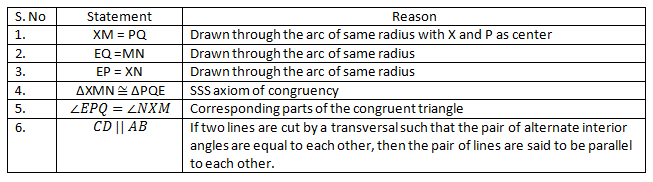# Construction Of Parallel Lines

Have you ever observed the railway tracks or the opposite edges of a door? One of the interesting facts about these is that they are examples of parallel lines. Now the question arises what exactly are parallel lines? The lines which do not have a common meeting point in the same plane, however far they are extended are known as parallel lines. The following figure represents parallel lines: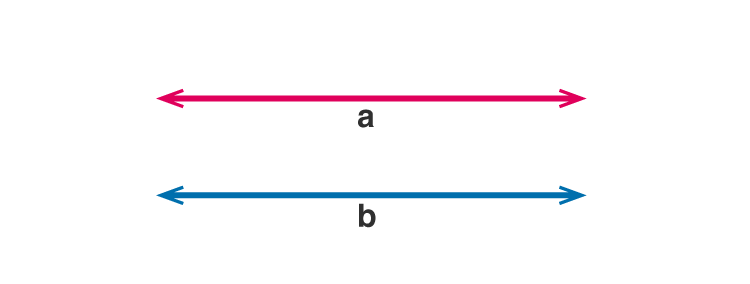Two lines are said to be parallel in a plane if they do not intersect if extended till infinite in both the directions. The distance between two lines is similar throughout the whole length. The symbol to denote the parallel lines is ||. Suppose the two lines ‘a’ and ‘b’ are parallel to each other, we can express their parallel nature using a symbol by a||b and read as ‘a is parallel to b’.

## Steps to Construct Parallel Lines

To construct a line parallel to the other line from an external point we require a ruler and a compass and the following steps are followed:

Given: A line segment AB and a point P that lies out of the line segment AB

To construct a line that is parallel to line AB that passes through point P.

• Step 1: Choose any point X on the line segment AB and join it to point P as shown.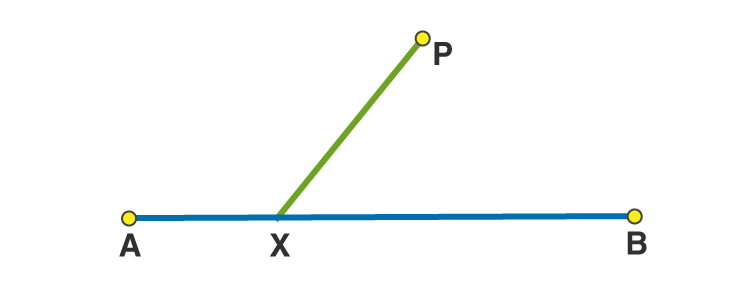• Step 2: With X as center and any suitable radius draw an arc cutting the line segment PX at point M and AB at point N respectively.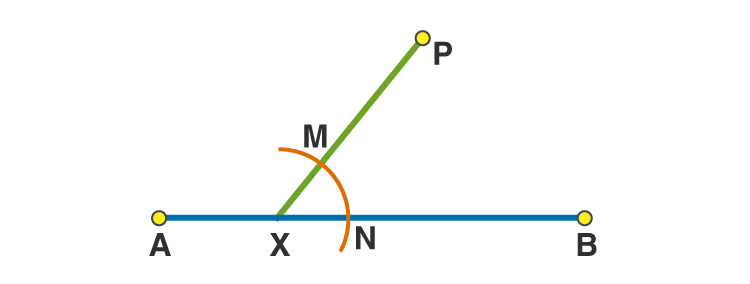• Step 3: With P as center and radius as used in the previous step draw an arc EF cutting the line segment PX at point Q as shown.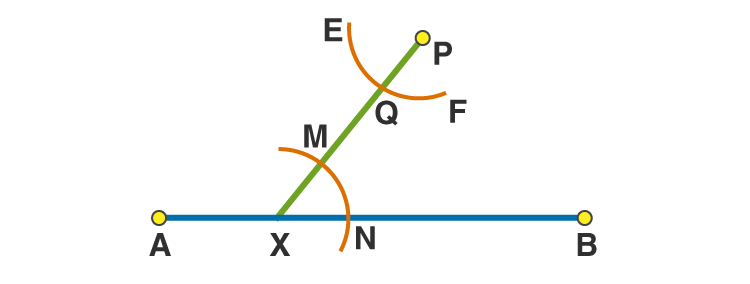• Step 4: With Q as center and same radius as before, draw an arc cutting the arc EF at R as shown below.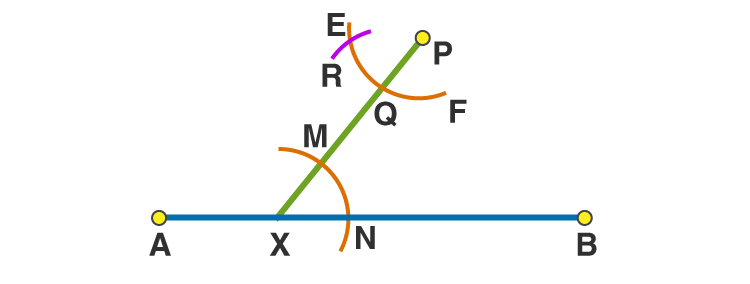• Step 5: Join R and P to draw a line segment CD as shown.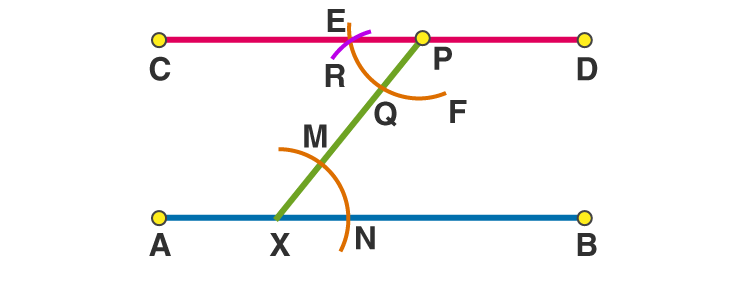The line segment CD is the required line parallel to the line segment AB and passes through the point P.

To verify whether the line segment that is constructed is parallel to line segment AB, connect the points E, Q and M, N as described below: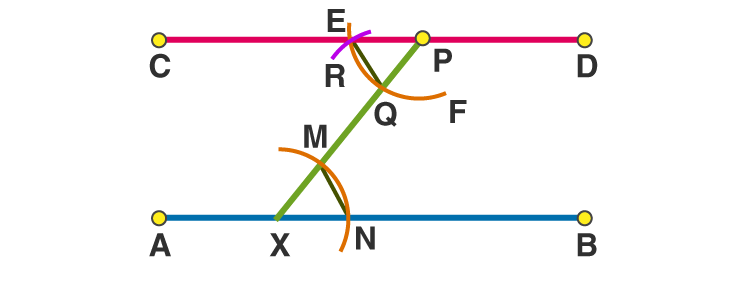In the triangle EPQ and XMN,